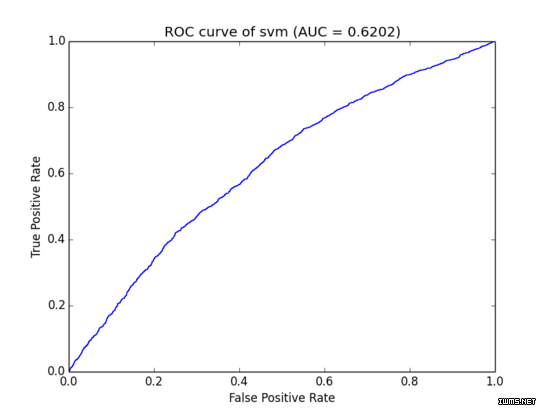# 使用Python画ROC曲线以及AUC值

9,081

### AUC介绍

`AUC` (Area Under Curve)是机器学习二分类模型中非常常用的评估指标，相比于 `F1-Score` 对项目的不平衡有更大的容忍性，目前常见的机器学习库中(比如 scikit-learn)一般也都是集成该指标的计算，但是有时候模型是单独的或者自己编写的，此时想要评估训练模型的好坏就得自己搞一个 `AUC` 计算模块，本文在查询资料时发现 `libsvm-tools` 1 有一个非常通俗易懂的 `auc` 计算，因此抠出来用作日后之用。

AUC计算

`AUC` 的计算分为下面三个步骤：

1. 计算数据的准备，如果模型训练时只有训练集的话一般使用交叉验证的方式来计算，如果有评估集( `evaluate` )一般就可以直接计算了，数据的格式一般就是需要预测得分以及其目标类别（注意是目标类别，不是预测得到的类别）
2. 根据阈值划分得到横（X: `False Positive Rate` ）以及纵（Y: `True Positive Rate` ）点
3. 将坐标点连成曲线之后计算其曲线下面积,就是 `AUC` 的值

```#! -*- coding=utf-8 -*-import pylab as pl
from math import log,exp,sqrtevaluate_result="you file path"db = []  #[score,nonclk,clk]pos, neg = 0, 0 with open(evaluate_result,'r') as fs:for line in fs:
nonclk,clk,score = line.strip().split('\t')
nonclk = int(nonclk)
clk = int(clk)
score = float(score)
db.append([score,nonclk,clk])pos += clk
neg += nonclk

db = sorted(db, key=lambda x:x, reverse=True)#计算ROC坐标点xy_arr = []
tp, fp = 0., 0.
for i in range(len(db)):
tp += db[i]
fp += db[i]
xy_arr.append([fp/neg,tp/pos])#计算曲线下面积auc = 0.
prev_x = 0for x,y in xy_arr:if x != prev_x:
auc += (x - prev_x) * yprev_x = xprint "the auc is %s."%aucx = [_v for _v in xy_arr]y = [_v for _v in xy_arr]
pl.title("ROC curve of %s (AUC = %.4f)" % ('svm',auc))
pl.xlabel("False Positive Rate")
pl.ylabel("True Positive Rate")
pl.plot(x, y)# use pylab to plot x and ypl.show()# show the plot on the screen```

`nonclk \t clk \t score`

1. `nonclick` :未点击的数据，可以看做负样本的数量
2. `clk` :点击的数量，可以看做正样本的数量
3. `score` :预测的分数，以该分数为group进行正负样本的预统计可以减少 `AUC` 的计算量### 注意

1. 只能计算二分类的结果（至于二分类的标签随便处理）
2. 上面代码中每个 `score` 都做了一次阈值，其实这样效率是相当低的，可以对样本进行采样或者在计算横轴坐标时进行等分计算
• 版权声明 本文源自 蓝桥杯 整理
• 转载请务必保留本文链接：https://www.plob.org/article/12514.html